Anúncio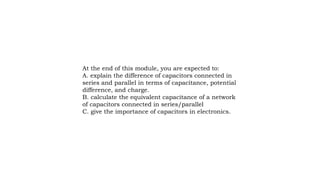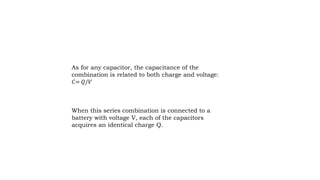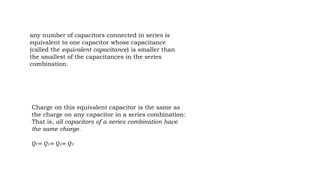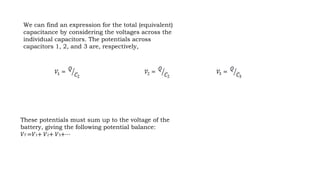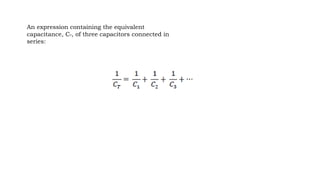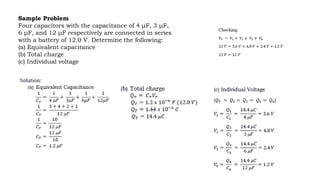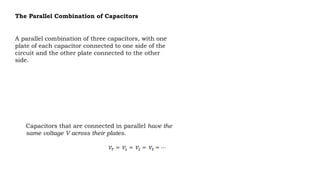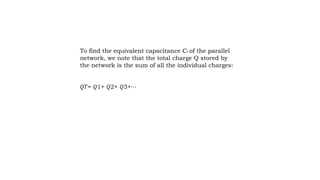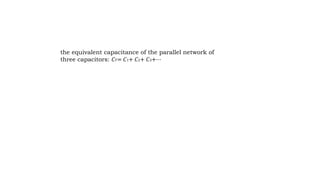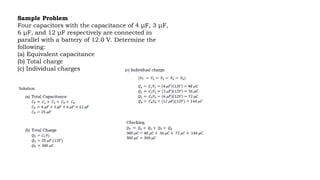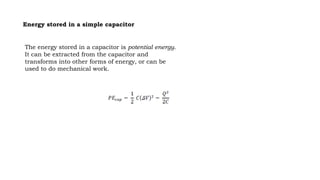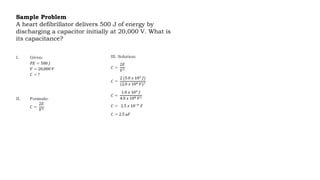1 de 13
Anúncio

### Series and Parallel Capacitors.pptx

1. Series and Parallel Capacitors
2. At the end of this module, you are expected to: A. explain the difference of capacitors connected in series and parallel in terms of capacitance, potential difference, and charge. B. calculate the equivalent capacitance of a network of capacitors connected in series/parallel C. give the importance of capacitors in electronics.
3. As for any capacitor, the capacitance of the combination is related to both charge and voltage: 𝐶= 𝑄/𝑉 When this series combination is connected to a battery with voltage V, each of the capacitors acquires an identical charge Q.
4. Charge on this equivalent capacitor is the same as the charge on any capacitor in a series combination: That is, all capacitors of a series combination have the same charge. 𝑄𝑇= 𝑄1= 𝑄2= 𝑄3 any number of capacitors connected in series is equivalent to one capacitor whose capacitance (called the equivalent capacitance) is smaller than the smallest of the capacitances in the series combination.
5. We can find an expression for the total (equivalent) capacitance by considering the voltages across the individual capacitors. The potentials across capacitors 1, 2, and 3 are, respectively, These potentials must sum up to the voltage of the battery, giving the following potential balance: 𝑉𝑇 =𝑉1+ 𝑉2+ 𝑉3+⋯
6. An expression containing the equivalent capacitance, CT, of three capacitors connected in series:
7. Sample Problem Four capacitors with the capacitance of 4 μF, 3 μF, 6 μF, and 12 μF respectively are connected in series with a battery of 12.0 V. Determine the following: (a) Equivalent capacitance (b) Total charge (c) Individual voltage
8. The Parallel Combination of Capacitors Capacitors that are connected in parallel have the same voltage V across their plates. A parallel combination of three capacitors, with one plate of each capacitor connected to one side of the circuit and the other plate connected to the other side.
9. To find the equivalent capacitance CT of the parallel network, we note that the total charge Q stored by the network is the sum of all the individual charges: 𝑄𝑇= 𝑄1+ 𝑄2+ 𝑄3+⋯
10. the equivalent capacitance of the parallel network of three capacitors: 𝐶𝑇= 𝐶1+ 𝐶2+ 𝐶3+⋯
11. Sample Problem Four capacitors with the capacitance of 4 μF, 3 μF, 6 μF, and 12 μF respectively are connected in parallel with a battery of 12.0 V. Determine the following: (a) Equivalent capacitance (b) Total charge (c) Individual charges
12. Energy stored in a simple capacitor The energy stored in a capacitor is potential energy. It can be extracted from the capacitor and transforms into other forms of energy, or can be used to do mechanical work.
13. Sample Problem A heart defibrillator delivers 500 J of energy by discharging a capacitor initially at 20,000 V. What is its capacitance?
Anúncio